Software Engineering
Volume 4, Issue 2, March 2016, Pages: 13-18

A Fast Method for Community Detection Based on Compressing Networks

Jialin He1, 2, Duanbing Chen1, 2, *, Chongjing Sun1, 2

1Web Sciences Center, University of Electronic Science and Technology of China, Chengdu, China

2Big Data Research Center, University of Electronic Science and Technology of China, Chengdu, China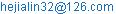(Jialin He)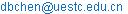(Duanbing Chen)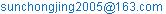(Chongjing Sun)

*Corresponding author

Jialin He, Duanbing Chen, Chongjing Sun. A Fast Method for Community Detection Based on Compressing Networks. Software Engineering. Vol. 4, No. 2, 2016, pp. 13-18. doi: 10.11648/j.se.20160402.12

Received: March 31, 2016; Accepted: April 8, 2016; Published: April 16, 2016

Abstract: Community structure is one of important characteristics in complex networks and contributes to the understanding of function in corresponding complex systems. Many methods based on modularity optimization have been proposed in the past few years. To obtain a good community partition, they must maximize the modularity from scratch, which havelow efficiency.In this paper, we suggest a novel method which first constructs a small network according to connection strength index and then uses Blondel method to detect communities. Experimental results on real and synthetic networks show that compared with the traditional method our method can not only maintain the quality of communities but also improve the efficiency greatly.

Keywords: Complex Networks, Community Structure, Modularity Optimization, Connection Strength, Small Network

Contents

1. Introduction

Complex network, in its simple form, is a set of nodes joined in pairs by edges. Many complex systems can be represented by networks [1-4]. Examples include Internet , in which the nodes are computers and the edges are data connections such as optical-fibre cables, co-authorship networks , in which the nodes are authors and the edges are co-authorships between authors, and social networks , in which the nodes are people and the edges represent social interactions. Community structure [8-11] is an important characteristic of network whose nodes are more tightly connected with each other than with other nodes in the network. In a social network, a community might correspond to an actual community in real world, a group of people brought together by common interest, common location or workplace, or family ties .

In the past few years, many methods based on modularity optimization are suggested to detect communities in complex network. Guimera et al.  showed that finding the modularity of a network is analogous to finding the ground-state energy of a spin system. The method combines two types of "moves": local moves, where a single node is shifted from one cluster to another, taken at random; global moves, consisting of mergers and splits of communities. Duch et al.  proposed a method to find the community structure in complex networks based on an extremal optimization of the value of modularity. The method outperforms the optimal modularity found by the existing algorithms in the literature giving a better understanding of the community structure. Newman et al.  described a new algorithm which gave excellent results when tested on both computer-generated and real-world networks. However, the time complexity of this algorithm is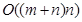, oron a sparse network. Clauset et al.  presented a hierarchical agglomeration algorithm for detecting community structure which is faster than many competing algorithms. Its running time on a network with n nodes and m edges iswhere d is the depth of the dendrogram describing the community structure. Medus et al.  presented an analysis of community structure in networks based on the application of simulated annealing techniques. They compared their method with other methodologies based on betweenness analysis and showed that in all cases a better community structure can be attained. Chen et al.  suggested an overlapping community detection algorithm which first finds an initial partial community from a node with maximal node strength and then adds tight nodes to expand the partial community. Guo et al.  presented a novel method to detect the communities in un-weighted, weighted, un-directed, directed and signed networks by constructing a dissimilarity distance matrix of network and identifying community centers with maximizing modularity. Dinh et al.  introduced an adaptive framework with approximation guarantees for quickly identifying community structure in complex networks via maximizing modularity Q. Their framework explores the advantages of power-law distribution property found in many real-world complex systems. Chen et al.  proposed a fast and efficient algorithm for detecting community structures in complex networks. The key strategy of the algorithm is to mine a node with the closest relations with the community and assign it to this community. Agarwal et al.  showed that the technique of rounding solutions to fractional mathematical programs yields high-quality modularity maximizing communities, while also providing a useful upper bound on the best possible modularity. The drawback of their algorithm is their resource requirement. Due to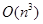constraints in the linear program, andvariables in the quadratic program, the algorithm currently does not scale beyond about 300 and 4000 nodes respectively. However, all these algorithms have a drawback: they must maximize the modularity from scratch, which have low efficiency.

In this paper, we suggest a novel method which first constructs a small network according to connection strength index and then detects communities on the small network. Experimental results on real and synthetic networks show that by constructing a small network, our method can not only maintain the quality of communities but also improve efficiency.

2. Method

Let G=(V,E) be an undirected network with node set V={v1,…,vn}.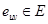indicates a connection between the nodes u and v. The neighborhood of a nodeis the set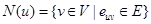. The notation wuv represents the weight of an edge euv. For an unweighted network, the weight wuv of any edge euv is equal to 1.

2.1. Blondel Method

The Blondel method  is a fast community detection method which is composed of two steps. Initially, each node in network is considered as a community. Then, for each node u, one considers the neighbor v of u and computes the gain of modularity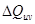by putting u into the community which contains node v temporarily. The node u is then placed in the community of its neighbor that yields the largest gain of modularity, as long as it is positive. Theis computed by(1)

where ku,v is the sum of weights of the edges from u to nodes in community Cv, ku,u is the sum of weights of the edges from u to nodes in community Cu, ku is the sum of the weights of the edges incident to node u, kC(u) is the sum of the weights of the edges inside community Cu, kC(v) is the sum of the weights of the edges inside community Cv and wG is the sum of the weights of all the edges in the network. The first step is iterated until all nodes can’t move. In the second step, each community is considered as a super node, and the weight of the edge between two super nodes are given by the sum of the weights of the edges between two corresponding communities at the lower level. The two steps are repeated until there is no more improvement and maximal modularity is achieved. The computational time grows like O(m), where m is the number of edges in network, so the algorithm is extremely fast.

2.2. Connection Strength Index

For an undirected and unweighted network, the connection strength of two connected nodes u and v is defined as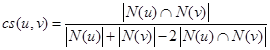(2)

where two sets N(u) and N(v) are the neighbors of two nodes u and v respectively. If two nodes u and v have the same neighbors, the value cs(u,v) is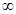. A very small constant is added in the denominator to avoid cs(u,v) being. If two nodes u and v has no common neighbors, the cs(u,v) is equal to 0. So the larger the value cs(u,v) is, the stronger the connection of two connected nodes u and v is.

In Eq.(2), most of time is consumed in the computation of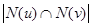. To compute the valueintime, the counting sort method  is used. Assume that there is an auxiliary array C with n elements, all initialized to 0. We first make one pass through the set N(v) and for each nodethat we see, we increment C[p] by 1. Then we sweep each node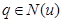and count the number of C[q]=1, i.e., the value.

2.3. Compressing and Partitioning

Given an undirected and unweighted network G(V,E), we can obtain an initial community division by taking advantage of connection strength index and then construct a small network based on these initial communities. The Blondel method is used to detect final communities on this small network. The details are described as follows:

(i) Compute the connection strength for all pairs of connected nodes by Eq.(2).

(ii) First, all edges are removed from network G(V,E). Then, given a node u, if,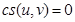, do nothing; if,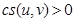, an edge is added between node u and the neighbor v with the largest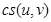.

(iii) If a node has no edges, it is still a single node in the small network. If two or more nodes are connected, they are considered as a node in the small network. In the small network, all nodes are called ‘super node’ which contains one or more nodes. Two super nodes are connected if there is at least an edge between them in the original network. The weight of a new edge is the number of the edges between two super nodes in original network.

(iv) The Blondel method is used to detect communities on the small network.Figure 1. The community detection process of our method. (a) A toy network with two communities; (b) the initial community partition according to connection strength index; (c) the construction of a small network; (d) the final communities.

We use a toy network with two communities to illustrate our method, as shown in Figure 1. First, all edges in Figure 1(a) are removed. Then for node 5, because of cs(5,6)=0 and cs(5,7)=0, it has no edges and is still a single node in the small network. For node 6, the cs(6,1) is the largest among its three neighbors, so the edge e16 is added between two nodes 1 and 6. Similarly, the edge between two nodes 1 and 2 is added. Three nodes 1, 2 and 6 are constructed as a super node in the small network because they are connected. The node 1 is chosen as the super node id. Other nodes are processed in a similar way and the results are shown in Figure 1(b)-(d).

2.4. Computational Complexity

Our method is composed of three parts: the computation of each edge’s connection strength, the construction of a small network and the community detection. For the computation of one edge’s connection strength, the time complexity is O(1), so m edges take O(m) time. For the construction of a small network, the breadth-first search (BFS)  is used to find initial communities, so the part takes O(n) time. Because of using the Blondel method, the time complexity of community detection is O(m’), where m’ is the number of edges in small network. Since m’<<m and n<m in real networks, the computational complexity of our algorithm is O(m).

3. Experimental Results

To evaluate the performance of our method (labeled as CBlondel), we compare it with the traditional strategy (labeled as Blondel), i.e., the communities are detected from scratch by using the Blondel method. Two methods have been compiled and tested on the laptop with a core i7-4702 MQ and 8 GB memory. In the implementation, two methods share the same the Blondel method. The only difference is that our method constructs a small network before using the Blondel method.

3.1. Real Networks

Gowalla  contains user-user friendship relations. Nodes represent users and an edge indicates a friendship between two users. Dblp  is a co-authorship network of DBLP computer science bibliography. Nodes are authors and an edge between two nodes exists if the corresponding authors have published at least one paper together. Youtube  is a social network from a video-sharing web site. Users form friendships with each other and users can create groups in which other users can join. In the network, nodes represent users and an edge between two nodes indicates a friendship. Stanford  is the hyperlink network of the websites of the universities in Berkley and Stanford. Nodes represent web pages and edges represent hyperlinks. The detailed information of four real networks is listed in Table 1.

Table 1. The topological properties of four real networks, including the number of nodes, the number of edges, average degree (<k>), mean squared degree (<k2>), global clustering coefficient (cc), power law exponent (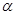).

 network #nodes #edges cc α Gowalla 196591 950327 9.67 2964.03 0.024 2.65 Dblp 317080 1049866 6.62 144.01 0.306 3.26 Stanford 654782 6581871 20.10 65804.78 0.009 2.60 Youtube 1134890 2987624 5.26 2603.72 0.006 2.14

Table 2 shows the experimental results of two methods. From Table 2, it can be seen that compared with the traditional method our method maintains the quality of communities in four real networks. In Blondel method, the modularity depends on the sweeping order of nodes. However, the sweeping order of nodes does not have a significant influence on the modularity. Because of constructing a small network, the sweeping order of nodes for our method is different from that for the traditional one. So the modularity of our method is slightly higher than the traditional one on Gowalla, Dblp and Youtube networks, but slightly lower than the traditional one on Stanford network.

Meanwhile, Table 2 shows that the running time of our method is faster than that of the traditional one on four real networks. For the traditional method, to maximize the modularity gain in the first iteration, each edge is computed many times. However, for our method, each edge is computed only once. So compared with the traditional method, the coefficient of time complexity for our method is smaller. To quantify the efficiency of our method, an index called "speedup ratio" is defined(3)

where Tour is the CPU running time for our method and Ttraditional for the traditional one. On Gowalla and Dblp networks, the speedup ratio is less than 45%. However, on Youtube and Stanford networks, the speedup is more than 65%. So the speedup ratio is related with the network structure.

Table 2. The experimental results on four real networks, including the CPU running time(s), modularity (Q) and speedup ratio.

 network modularity running time (s) Speedup ratio Blondel CBlondel Blondel CBlondel Gowalla 0.687 0.697 15.88 9.25 0.42 Dblp 0.805 0.818 12.76 8.80 0.31 Stanford 0.937 0.932 311.53 54.44 0.82 Youtube 0.685 0.707 218.38 72.95 0.66

3.2. Synthetic Networks

We also test the performance of our method on some synthetic scale-free networks which are generated by LFR model . In the LFR model, both the degree and the community size distributions are power laws, with exponents α and β, respectively. The synthetic scale-free networks are built as follows:

1. Each node is given a degree taken from a power law distribution with exponent α. The extremes of the distribution kmin and kmax are chosen such that the average degree is <k>. The configuration model  is used to connect the nodes to keep their degree sequence.

2. Each node shares a fraction 1-μ of its edges with the other nodes of its community and a fraction μ with the nodes of the other communities: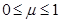is the mixing parameter.

3. The sizes of the communities are taken from a power law distribution with exponent β, such that the sum of all sizes equals the number of nodes of the network. The minimal and maximal community sizes smin and smax are chosen to respect the constraints imposed by our definition of community: smin>kmin and smax>kmax. This ensures that a node of any degree can be included in at least a community.

4. At the beginning, all nodes are homeless, i.e., they are not assigned to any community. In the first iteration, a node is assigned to a randomly chosen community; if the community size exceeds the internal degree of the node (i.e., the number of its neighbors inside the community), the node enters the community, otherwise it remains homeless. In successive iterations we place a homeless node to a randomly chosen community: if the latter is complete, we kick out a randomly selected node of the community, which becomes homeless. The procedure stops when there are no more homeless nodes.

5. To enforce the condition on the fraction of internal neighbors expressed by the mixing parameter μ, several rewiring steps are performed, such that the degrees of all nodes stay the same and only the split between internal and external degree is affected, when needed. In this way the ratio between external and internal degree of each node in its community can be set to the desired share μ with good approximation.

First, we generate four synthetic networks with the same parameters α and β, which are set to 2.5 and 1.5 respectively. The only difference for four synthetic networks is the mixing parameters μ, which is set to 0.1, 0.2, 0.3 and 0.4, respectively. The smaller the mixing parameter μ is, the stronger the community structure is. The detailed information of four synthetic networks is described in Table 3.

Table 3. The topological properties of four synthetic networks with different community structure, including the number of nodes, the number of edges, average degree (<k>), global clustering coefficient (cc).

 network #nodes #edges kmin α β μ cc LFR1 500000 3242458 5 13.0 2.5 1.5 0.1 0.041 LFR2 500000 3242458 5 13.0 2.5 1.5 0.2 0.030 LFR3 500000 3242458 5 13.0 2.5 1.5 0.3 0.021 LFR4 500000 3242458 5 13.0 2.5 1.5 0.4 0.015

From Table 4, it can be seen that our method not only maintains the quality of communities but also improves efficiency in all cases compared with the traditional one. Forand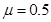, the speedup ratio is more than 10%. For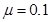and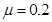, the speedup ratio is more than 35%. These results also show that our method has to do with the community structure. For strong community structure, the size of small network is really small because many connected nodes have common neighbors. However, for weak community structure, the size of small network is still large because many connected nodes have no common neighbors. From above analysis, our method is suitable for the networks with strong community structure.

Table 4. The experimental results on four synthetic networks with different community structure, including the CPU running time(s), modularity (Q) and speedup ratio.

 network modularity running time (s) Speedup ratio Blondel CBlondel Blondel CBlondel LFR1 0.846 0.846 48.50 29.77 0.39 LFR2 0.770 0.769 57.01 35.12 0.38 LFR3 0.670 0.670 56.33 46.65 0.17 LFR4 0.575 0.580 49.92 44.88 0.10

Second, we generate four synthetic scale-free networks with different number of communities. As shown in Table 5, all the parameters of four synthetic networks are the same. In order to generate different number of communities, the number of nodes for four synthetic networks is different. From Table 6, it can be seen that with the increase of the number of communities, the speedup ratio has only small changes. So the number of communities has little influence on speedup ratio.

Table 5. The topological properties of four synthetic networks with different number of communities, including the number of nodes, the number of edges, average degree (<k>), the number of communities (nc).

 network #nodes #edges kmin α β μ nc LFRC1 300000 1944393 5 13.0 2.5 1.5 0.1 456 LFRC2 350000 2267290 5 13.0 2.5 1.5 0.1 528 LFRC3 500000 3238714 5 13.0 2.5 1.5 0.1 733 LFRC4 550000 3563300 5 13.0 2.5 1.5 0.1 843

Table 6. The experimental results on four synthetic networks with different number of communities, including the CPU running time(s), modularity (Q) and speedup ratio.

 network modularity running time (s) Speedup ratio Blondel CBlondel Blondel CBlondel LFRC1 0.844 0.844 28.58 18.79 0.34 LFRC2 0.845 0.846 29.78 20.22 0.32 LFRC3 0.847 0.846 47.63 32.75 0.31 LFRC4 0.846 0.846 47.51 34.15 0.28

Finally, we generate four synthetic networks with different parameters α and β. In LFR model, two parameters α and β take typical values:,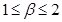. To evaluate the impact of two parameters on our method, we generate two groups of synthetic networks: (1) when parameter α is set to 2.5, the parameter β is set to 1.2 and 1.8 respectively; (2) when parameter β is set to 1.5, the parameter α is set to 2.2 and 2.8 respectively. The detailed information of four synthetic networks is described in Table 7.

Table 7. The topological properties of four synthetic networks with different exponent parameters, including the number of nodes, the number of edges, average degree (<k>), global clustering coefficient (cc).

 network #nodes #edges kmin α β μ cc LFRP1 500000 3257667 6 13.0 2.5 1.2 0.1 0.101 LFRP2 500000 3257321 6 13.0 2.5 1.8 0.1 0.118 LFRP3 500000 3262103 6 13.0 2.2 1.5 0.1 0.118 LFRP4 500000 3240603 6 13.0 2.8 1.5 0.1 0.063

The experimental result is shown in Table. 8. When parameter, the speedup ratio is 30% for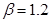and 32% for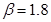. So the parameter β has little impact on the speedup ratio. The reason is that different β only leads to different number of communities. When parameter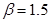, the speedup ratio is 33% forand 22% for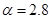. So the parameter α is related to the speedup ratio. The reason can be explained as follows. The larger the parameter α is, the more the small degree of nodes is. However, for large α, to generate a network with designated average degree <k>, the LFR model must generate many nodes with larger degree which lead to small global clustering coefficient (cc). From Table 7, it can be seen that the cc is 0.118 forand 0.063 for. For our method, the small cc will results in large size of small network because many connected nodes have less common neighbors. So our method is suitable for the network with small parameter α.

Table 8. The experimental results on four synthetic networks with different exponent parameters, including the CPU running time(s), modularity (Q) and speedup ratio.

 network modularity running time (s) Speedup ratio Blondel CBlondel Blondel CBlondel LFRP1 0.857 0.857 42.17 29.44 0.30 LFRP2 0.848 0.848 44.47 30.18 0.32 LFRP3 0.866 0.866 46.10 30.84 0.33 LFRP4 0.845 0.845 43.19 33.44 0.22

4. Conclusion

In this paper, we suggest a novel method which first constructs a small network according to connection strength index and detects communities on the small network by using Blondel method. According to connection strength index, a node may have no edge or at least one edge. If a node has no edge, it is still a node in the small network. If many nodes are connected, they are considered as a node in the small network.

The performance of our method is evaluated on real and synthetic networks. First, on four real networks, our method maintains the quality of communities compared with the traditional one. Meanwhile, our method is always faster than the traditional one. Second, on four synthetic networks with different community structure, experimental results show that our method is suitable for the networks with strong community structure. Third, on four synthetic networks with different number of communities, experimental results show that the number of communities has little influence on our method. Finally, on four synthetic networks with different parameters α and β, experimental results show that our method is suitable for the network with small parameter α.

There are two open issues needing further study in the future. First, apart from community structure, the number of communities and exponent parameters, there are many other network characteristics which have an influence on the speed ratio. Much work is needed to find them in the future. Second, with the available of temporal data in recent years, the community detection in temporal networks has caused great concern . So the further research on the community detection in temporal networks is needed.

Acknowledgements

This work is partially supported by the National Natural Science Foundation of China under Grant No. 61433014 and by the Fundamental Research Funds for the Central Universities under Grant No. ZYGX2014Z002.

References

1. Albert R, Barabási A L. Statistical mechanics of complex networks [J]. Reviews of Modern Physics, 2002, 74(1): 47.
2. Dorogovtsev S N, Mendes J F F. Evolution of networks [J]. Advances in Physics, 2002, 51(4): 1079-1187.
3. Boccaletti S, Latora V, Moreno Y, et al. Complex networks: Structure and dynamics [J]. Physics Reports, 2006, 424(4): 175-308.
4. Newman M E J. The structure and function of complex networks [J]. SIAM Review, 2003, 45(2): 167-256.
5. Faloutsos M, Faloutsos P, Faloutsos C. On power-law relationships of the internet topology [C]// ACM SIGCOMM Computer Communication Review. ACM, 1999, 29(4): 251-262.
6. Newman M E J. The structure of scientific collaboration networks [J]. Proceedings of the National Academy of Sciences, 2001, 98(2): 404-409.
7. Zachary W W. An information flow model for conflict and fission in small groups [J]. Journal of Anthropological Research, 1977: 452-473.
8. Girvan M, Newman M E J. Community structure in social and biological networks [J]. Proceedings of the National Academy of Sciences, 2002, 99(12): 7821-7826.
9. Fortunato S. Community detection in graphs [J]. Physics Reports, 2010, 486(3): 75-174.
10. Malliaros F D, Vazirgiannis M. Clustering and community detection in directed networks: A survey [J]. Physics Reports, 2013, 533(4): 95-142.
11. Porter M A, Onnela J P, Mucha P J. Communities in networks [J]. Notices of the AMS, 2009, 56(9): 1082-1097.
12. Newman M E J. Communities, modules and large-scale structure in networks [J]. Nature Physics, 2012, 8(1): 25-31.
13. Guimera R, Sales-Pardo M, Amaral L A N. Modularity from fluctuations in random graphs and complex networks [J]. Physical Review E, 2004, 70(2): 025101.
14. Duch J, Arenas A. Community detection in complex networks using extremal optimization [J]. Physical Review E, 2005, 72(2): 027104.
15. Newman M E J. Fast algorithm for detecting community structure in networks [J]. Physical review E, 2004, 69(6): 066133.
16. Clauset A, Newman M E J, Moore C. Finding community structure in very large networks [J]. Physical review E, 2004, 70(6): 066111.
17. Medus A, Acuna G, Dorso C O. Detection of community structures in networks via global optimization [J]. Physica A: Statistical Mechanics and its Applications, 2005, 358(2): 593-604.
18. Chen D, Shang M, Lv Z, et al. Detecting overlapping communities of weighted networks via a local algorithm [J]. Physica A: Statistical Mechanics and its Applications, 2010, 389(19): 4177-4187.
19. Guo W F, Zhang S W. A general method of community detection by identifying community centers with affinity propagation [J]. Physica A: Statistical Mechanics and its Applications, 2016, 447: 508-519.
20. Dinh T N, Nguyen N P, Alim M A, et al. A near-optimal adaptive algorithm for maximizing modularity in dynamic scale-free networks [J]. Journal of Combinatorial Optimization, 2015, 30(3): 747-767.
21. Chen D, Fu Y, Shang M. A fast and efficient heuristic algorithm for detecting community structures in complex networks [J]. Physica A: Statistical Mechanics and its Applications, 2009, 388(13): 2741-2749.
22. Agarwal G, Kempe D. Modularity-maximizing graph communities via mathematical programming [J]. The European Physical Journal B, 2008, 66(3): 409-418.
23. Blondel V D, Guillaume J L, Lambiotte R, et al. Fast unfolding of communities in large networks [J]. Journal of statistical mechanics: theory and experiment, 2008, 2008(10): P10008.
24. Cormen T H. Introduction to algorithms [M]. MIT press, 2009.
25. Cho E, Myers S A, Leskovec J. Friendship and mobility: user movement in location-based social networks [C]//Proceedings of the 17th ACM SIGKDD International Conference on Knowledge Discovery and Data Mining. ACM, 2011: 1082-1090.
26. Ley M. The DBLP computer science bibliography: Evolution, research issues, perspectives [C]//String Processing and Information Retrieval. Springer Berlin Heidelberg, 2002: 1-10.
27. Yang J, Leskovec J. Defining and evaluating network communities based on ground-truth [J]. Knowledge and Information Systems, 2015, 42(1): 181-213.
28. Leskovec J, Lang K J, Dasgupta A, et al. Statistical properties of community structure in large social and information networks [C] //Proceedings of the 17th International Conference on World Wide Web. ACM, 2008: 695-704.
29. Lancichinetti A, Fortunato S, Radicchi F. Benchmark graphs for testing community detection algorithms [J]. Physical review E, 2008, 78(4): 046110.
30. Molloy M, Reed B. The size of the giant component of a random graph with a given degree sequence [J]. Combinatorics, Probability and Computing, 1998, 7(03): 295-305.
31. He J, Chen D. A fast algorithm for community detection in temporal network [J]. Physica A: Statistical Mechanics and its Applications, 2015, 429: 87-94.

 Contents 1. 2. 2.1. 2.2. 2.3. 2.4. 3. 3.1. 3.2. 4.
Article ToolsAbstractPDF(331K)